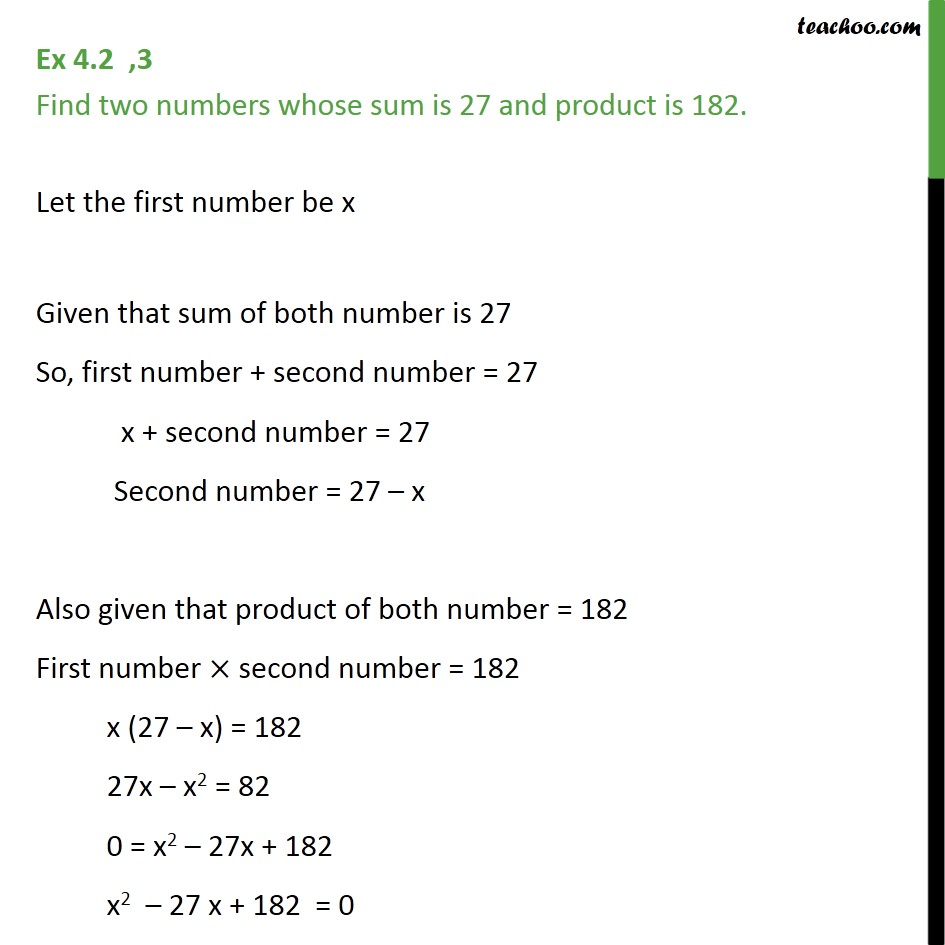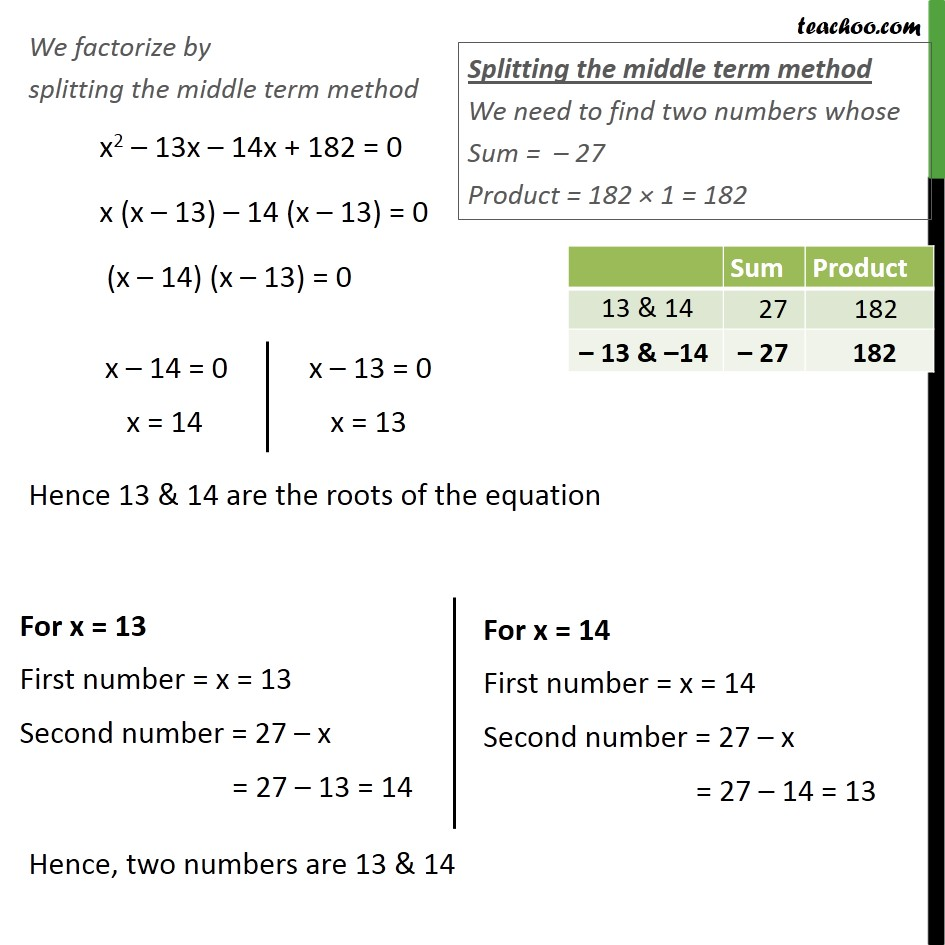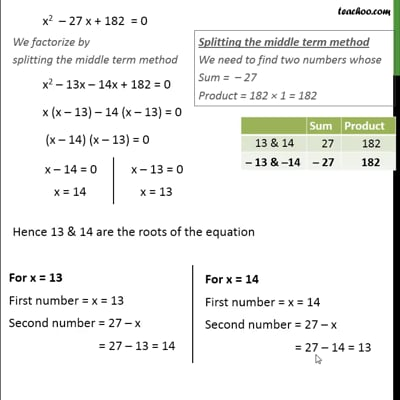Ex 4.2

Chapter 4 Class 10 Quadratic Equations
Serial order wiseThis video is only available for Teachoo black users

Introducing your new favourite teacher - Teachoo Black, at only ₹83 per month

### Transcript

Ex 4.2 ,3 Find two numbers whose sum is 27 and product is 182. Let the first number be x Given that sum of both number is 27 So, first number + second number = 27 x + second number = 27 Second number = 27 – x Also given that product of both number = 182 First number × second number = 182 x (27 – x) = 182 27x – x2 = 82 0 = x2 – 27x + 182 x2 – 27 x + 182 = 0 We factorize by splitting the middle term method x2 – 13x – 14x + 182 = 0 x (x – 13) – 14 (x – 13) = 0 (x – 14) (x – 13) = 0 Hence 13 & 14 are the roots of the equation Hence, two numbers are 13 & 14